Function Repository Resource:

# OrderedGraphModelPlot

Plots ordered trivalent graphs

Contributed by: Wolfram Physics Project Team
 ResourceFunction["OrderedGraphModelPlot"][triples] plots an ordered trivalent graph represented by the list of ordered triples triples.

## Details and Options

In the list triples, each triple corresponds to a vertex, and each number in each triple specifies the destination in the whole list of a particular edge. "Dangling connections" are represented by objects of the form h[number].
For the graph produced by ResourceFunction["OrderedGraphModelPlot"], each normal vertex is represented by a three-colored disk, while "dangling connections" are shown as gray circles.
ResourceFunction["OrderedGraphModelPlot"] takes the same options as Graph.

## Examples

### Basic Examples (1)

A simple ordered trivalent graph:

 In:=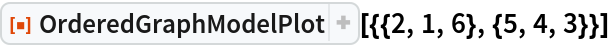Out=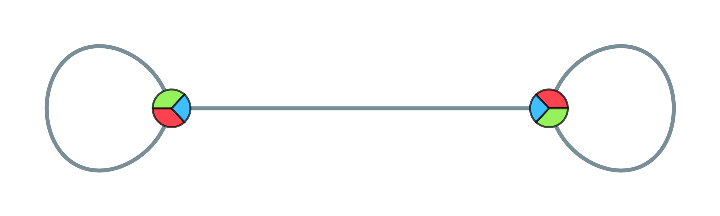### Scope (2)

Plot a simple ordered trivalent graph:

 In:=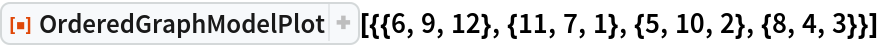Out=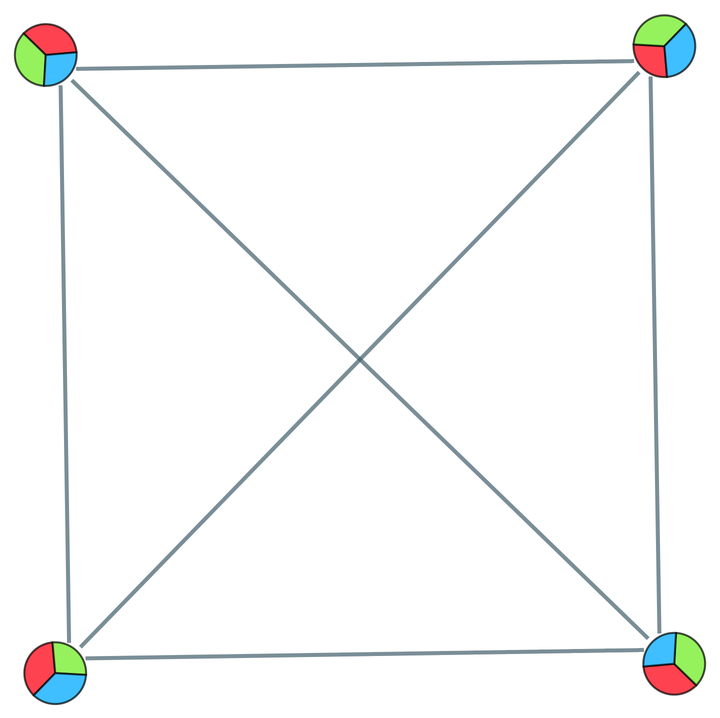Plot a simple ordered trivalent graph with dangling connections:

 In:=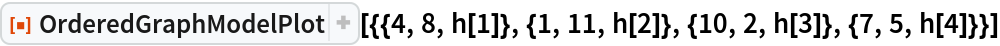Out=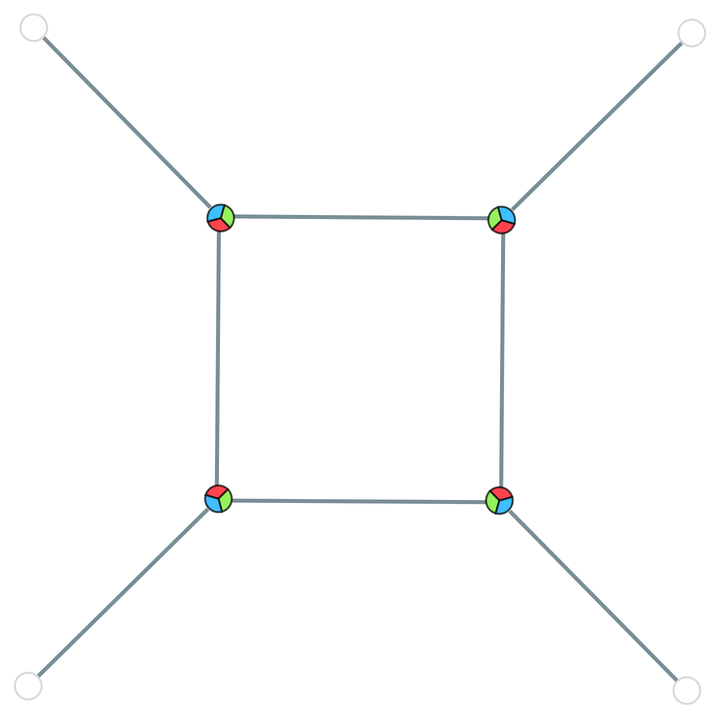## Version History

• 3.0.0 – 10 April 2020
• 2.0.0 – 08 April 2020
• 1.0.0 – 23 March 2020